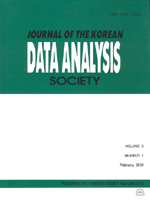상세검색
최근 검색어 전체 삭제
다국어입력
즐겨찾기0KCI등재 학술저널

# Simultaneous Tests for the Mean and Variance under the Normality

• 등재여부 : KCI등재
• 2015.06
• 1155 - 1164 (10 pages)

In this study, we propose likelihood ratio simultaneous test for the mean and variance under the scheme of the two-sample problem when the underlying distribution is normal. Then we consider the union-intersection test given by combining the jointly likelihood ratio statistics based on the approach proposed by Roy (1957). Here, we consider to use the combining functions to combine individual tests for each sub-null hypothesis under the union-intersection approach. Since it is difficult to derive the null distributions to compute the p-values for the tests considered in this study including the partial tests, we apply the permutation principle. Then we compare the efficiency among the proposed tests through a simulation study under the various sampling and changes of values of two parameters schemes. Moreover, we discuss some interesting features related to the likelihood ratio principle and the simultaneous test as concluding remarks. Finally, we comment briefly on the weighted combining function and applications of the permutation principle.

1. Introduction

2. Likelihood ratio test and some related ones

3. A simulation study

4. Some concluding remarks

References